# Angle

## Lower Key Stage 2

### Meaning

An angle is the space between two straight lines that cross each other.

Angles are labelled between two lines that cross over.
The angles inside a square or rectangle are described as right angles.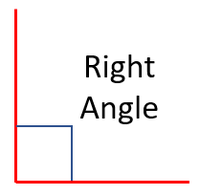A diagram showing a right angle between two lines. There are 4 right angles in a square or a rectangle.
An angle that is bigger than a right angle is called obtuse.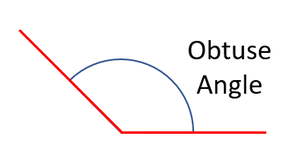This angle is bigger than a right angle so it is obtuse.
An angle that is smaller than a right angle is called acute.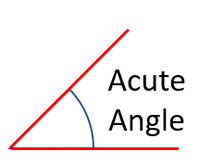This angle is smaller than a right angle so it is acute.

## Upper Key Stage 2

### Meaning

An angle is the space between two straight lines that cross each other.

Angles are measured in degrees (°).
A protractor can be used to measure the angle between to lines.
There are 360 degrees (360°) around a point.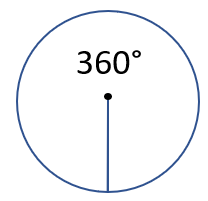The total angle around a point is 360°.
There are 90 degrees (90°) in a right angle.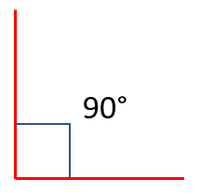A diagram showing that a right angle is 90°. There are 4 right angles in a square or a rectangle so the total angles inside added together makes 360°
An angle that is bigger than 90° is called obtuse.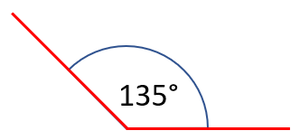This angle is bigger than 90° so it is obtuse.
An angle that is smaller than 90° is called acute.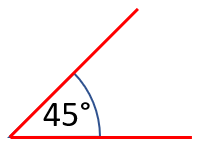This angle is smaller than a 90° so it is acute.
The angle on a straight line is 180°.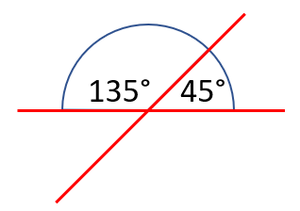A diagram showing 180° on a line. These two angles add up to 180° because they are part of a straight line.
A reflex angle is the angle on the opposite side of another angle.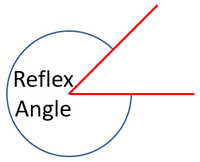An angle added to its reflex angle makes 360°.

## Key Stage 3

### Meaning

An angle is the space between two intersecting straight lines.

Angles are measured in degrees (°).
A protractor can be used to measure the angle between two intersecting lines.
There are 360 degrees (360°) around a point.The total angle around a point is 360°.
There are 90 degrees (90°) in a right angle.A diagram showing that a right angle is 90°. There are 4 right angles in a square or a rectangle so the total angles inside added together makes 360°
An angle that is bigger than 90° is called obtuse.This angle is bigger than 90° so it is obtuse.
An angle that is smaller than 90° is called acute.This angle is smaller than a 90° so it is acute.
The angle on a straight line is 180°.A diagram showing 180° on a line. These two angles add up to 180° because they are part of a straight line.
A reflex angle is the angle on the opposite side of another angle.An angle added to its reflex angle makes 360°.

## Key Stage 4

### Meaning

An angle is the space between two intersecting straight lines.

Angles are measured in degrees (°).
A protractor can be used to measure the angle between two intersecting lines.
There are 360 degrees (360°) around a point.The total angle around a point is 360°.
There are 90 degrees (90°) in a right angle.A diagram showing that a right angle is 90°. There are 4 right angles in a square or a rectangle so the total angles inside added together makes 360°
An angle that is bigger than 90° is called obtuse.This angle is bigger than 90° so it is obtuse.
An angle that is smaller than 90° is called acute.This angle is smaller than a 90° so it is acute.
The angle on a straight line is 180°.A diagram showing 180° on a line. These two angles add up to 180° because they are part of a straight line.
A reflex angle is the angle on the opposite side of another angle.An angle added to its reflex angle makes 360°.